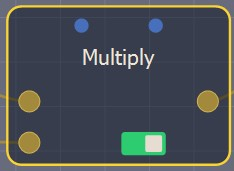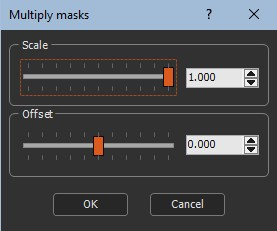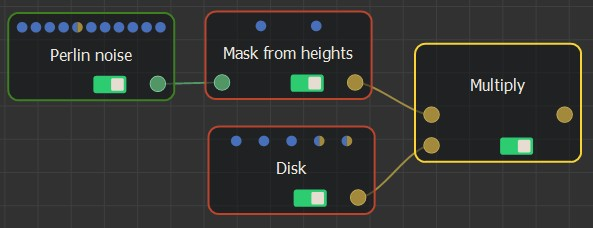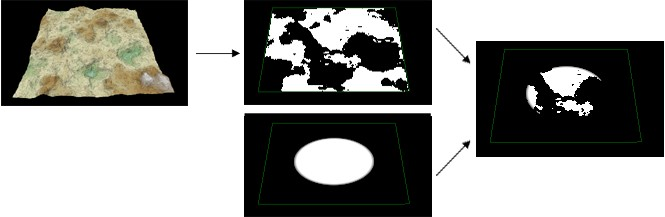### Adding a mask Multiply node

This node multiplies two input masks. At each vertex, the value of the output mask is the multiplication of the values of both input masks.

To add a node, right-click in the Graph Editor and select Create Node  > Mask Composition > Multiply.Double click on the node to open its parameters:### Editing a mask Multiply node

Edit the Multiply node in the parameters dialog:

• Scale: Sets the scale relative to zero.
• Offset: Adds or substracts a constant value to the resulting mask.

### Linking to input masks

Link the Multiply node to two input mask nodes.The maximum value for a mask is 1. At a given vertex, if the multiplication of the values of both masks exceeds 1, the output mask will have the value 1 at this vertex and not a value greater than 1. If both input masks do not have the same size or resolution, the size and resolution of the output mask is determined by the size and resolution of the first mask and the second mask is enlarged or decreased so that its size corresponds exactly to the size of the output mask, before being multiplied with the first mask.

For example, the mask from the heights of a Perlin noise node is multiplied with a disk node to give the output terrain shown below.### Parameters

Parameter Use
Scale Scale factor to be applied
Offset The offset (positive or negative) to apply

Copyright © 2021 · All Rights Reserved · Wysilab